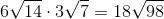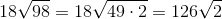## Example Questions

### Example Question #1 : How To Multiply Square Roots

Find the product: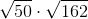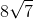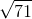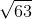Explanation: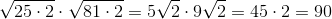### Example Question #1101 : Act Math

Simplify the following completely: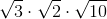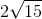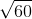Explanation:

To simplify this expression, simply multiply the radicands and reduce to simplest form.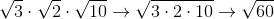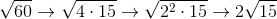### Example Question #3 : How To Multiply Square Roots

Simplify: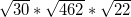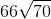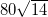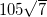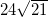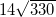Explanation:

When multiplying square roots, the easiest thing to do is first to factor each root. Thus: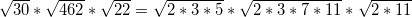Now, when you combine the multiplied roots, it will be easier to come to your final solution. Just multiply together everything "under" the roots: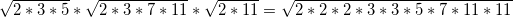Finally this can be simplified as: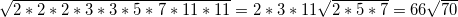### Example Question #4 : How To Multiply Square Roots

Simplify the following: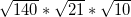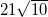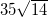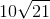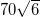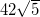Explanation:

When multiplying square roots, the easiest thing to do is first to factor each root. Thus: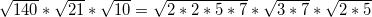Now, when you combine the multiplied roots, it will be easier to come to your final solution. Remember that multiplying roots is very easy! Just multiply together everything "under" the roots: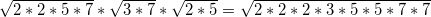Finally this can be simplified as: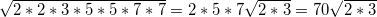### Example Question #5 : How To Multiply Square Roots

State the product: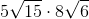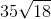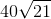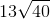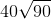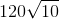Explanation: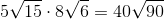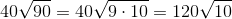### Example Question #6 : How To Multiply Square Roots

Find the product: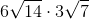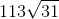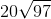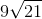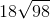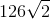Explanation: